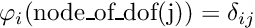GetFEM  5.4.2
getfem::fem_level_set Class Reference

FEM associated with getfem::mesh_fem_level_set objects. More...

#include <getfem_fem_level_set.h>

Inherits getfem::virtual_fem.

## Public Member Functions

void base_value (const base_node &x, base_tensor &t) const
Give the value of all components of the base functions at the point x of the reference element. More...

void grad_base_value (const base_node &x, base_tensor &t) const
Give the value of all gradients (on ref. More...

void hess_base_value (const base_node &x, base_tensor &t) const
Give the value of all hessians (on ref. More...

void real_base_value (const fem_interpolation_context &c, base_tensor &t, bool=true) const
Give the value of all components of the base functions at the current point of the fem_interpolation_context. More...

void real_grad_base_value (const fem_interpolation_context &c, base_tensor &t, bool=true) const
Give the gradient of all components of the base functions at the current point of the fem_interpolation_context. More...

void real_hess_base_value (const fem_interpolation_context &c, base_tensor &t, bool=true) const
Give the hessian of all components of the base functions at the current point of the fem_interpolation_context. More...Public Member Functions inherited from getfem::virtual_fem
virtual size_type nb_dof (size_type) const
Number of degrees of freedom. More...

virtual size_type nb_base (size_type cv) const
Number of basis functions.

size_type nb_base_components (size_type cv) const
Number of components (nb_dof() * dimension of the target space).

const std::vector< pdof_description > & dof_types () const
Get the array of pointer on dof description.

dim_type dim () const
dimension of the reference element.

dim_type target_dim () const
dimension of the target space.

vec_type vectorial_type () const
Type of vectorial element.

virtual bgeot::pconvex_ref ref_convex (size_type) const
Return the convex of the reference element.

bgeot::pconvex_structure basic_structure (size_type cv) const
Gives the convex of the reference element.

virtual const bgeot::convex< base_node > & node_convex (size_type) const
Gives the convex representing the nodes on the reference element.

bgeot::pconvex_structure structure (size_type cv) const
Gives the convex structure of the reference element nodes.

const base_node & node_of_dof (size_type cv, size_type i) const
Gives the node corresponding to the dof i. More...

bool is_lagrange () const
true if the base functions are such thatbool is_polynomial () const
true if the base functions are polynomials

template<typename CVEC , typename VVEC >
void interpolation (const fem_interpolation_context &c, const CVEC &coeff, VVEC &val, dim_type Qdim) const
Interpolate at an arbitrary point x given on the reference element. More...

template<typename MAT >
void interpolation (const fem_interpolation_context &c, MAT &M, dim_type Qdim) const
Build the interpolation matrix for the interpolation at a fixed point x, given on the reference element. More...

template<typename CVEC , typename VMAT >
void interpolation_grad (const fem_interpolation_context &c, const CVEC &coeff, VMAT &val, dim_type Qdim=1) const

template<typename CVEC , typename VMAT >
void interpolation_hess (const fem_interpolation_context &c, const CVEC &coeff, VMAT &val, dim_type Qdim) const
Interpolation of the hessian. More...

template<typename CVEC >
void interpolation_diverg (const fem_interpolation_context &c, const CVEC &coeff, typename gmm::linalg_traits< CVEC >::value_type &val) const
Interpolation of the divergence. More...

void add_node (const pdof_description &d, const base_node &pt, const dal::bit_vector &faces)
internal function adding a node to an element for the creation of a finite element method. More...

## Detailed Description

FEM associated with getfem::mesh_fem_level_set objects.

Definition at line 74 of file getfem_fem_level_set.h.

## ◆ base_value()

 void getfem::fem_level_set::base_value ( const base_node & x, base_tensor & t ) const
virtual

Give the value of all components of the base functions at the point x of the reference element.

Basic function used essentially by fem_precomp.

Implements getfem::virtual_fem.

Definition at line 79 of file getfem_fem_level_set.cc.

 void getfem::fem_level_set::grad_base_value ( const base_node & x, base_tensor & t ) const
virtual

Give the value of all gradients (on ref.

element) of the components of the base functions at the point x of the reference element. Basic function used essentially by fem_precomp.

Implements getfem::virtual_fem.

Definition at line 81 of file getfem_fem_level_set.cc.

## ◆ hess_base_value()

 void getfem::fem_level_set::hess_base_value ( const base_node & x, base_tensor & t ) const
virtual

Give the value of all hessians (on ref.

element) of the components of the base functions at the point x of the reference element. Basic function used essentially by fem_precomp.

Implements getfem::virtual_fem.

Definition at line 84 of file getfem_fem_level_set.cc.

## ◆ real_base_value()

 void getfem::fem_level_set::real_base_value ( const fem_interpolation_context & c, base_tensor & t, bool withM = true ) const
virtual

Give the value of all components of the base functions at the current point of the fem_interpolation_context.

Used by elementary computations. if withM is false the matrix M for non tau-equivalent elements is not taken into account.

Reimplemented from getfem::virtual_fem.

Definition at line 141 of file getfem_fem_level_set.cc.

 void getfem::fem_level_set::real_grad_base_value ( const fem_interpolation_context & c, base_tensor & t, bool withM = true ) const
virtual

Give the gradient of all components of the base functions at the current point of the fem_interpolation_context.

Used by elementary computations. if withM is false the matrix M for non tau-equivalent elements is not taken into account.

Reimplemented from getfem::virtual_fem.

Definition at line 168 of file getfem_fem_level_set.cc.

## ◆ real_hess_base_value()

 void getfem::fem_level_set::real_hess_base_value ( const fem_interpolation_context & c, base_tensor & t, bool withM = true ) const
virtual

Give the hessian of all components of the base functions at the current point of the fem_interpolation_context.

Used by elementary computations. if withM is false the matrix M for non tau-equivalent elements is not taken into account.

Reimplemented from getfem::virtual_fem.

Definition at line 200 of file getfem_fem_level_set.cc.

The documentation for this class was generated from the following files: